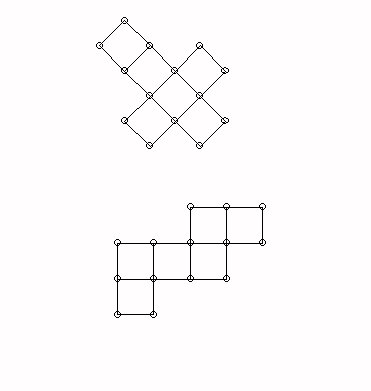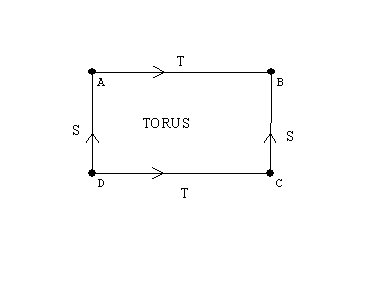# Monday  March 24

Discuss assignment for this week - make note of project and portfolio.

Review Classification of Isometries - Symmetries
An isometry is a transformation that preserves the distance between points.
We have discussed four isometries:
reflections, rotations, translations, and glide reflections.

The Product of isometries:
The product of two reflections is either a rotation (if the axes of the reflection intersect)  or a translation (if the axes of the reflection are parallel).

Wingeometry demonstration for reflection- one and two reflections

Any plane isometry  is either a reflection or  the product of two or three reflections.
Two reflections = rotation or translation.

Three reflections = reflection or glide reflection
Key idea- The product of two reflections is "flexible."

Space: How do we understand objects in space?
How can the Flatlander experience the sphere and space?

The simplest three dimensional figure has 4 points not all in the same plane: three point determine a plane- so a fourth point not in that plane will need "space" to make sense. These four points determine a tetrahedron.

Fold downs- flattened figures:
Consider how the tetrahedron can be assembled from folded down triangles.

Consider how the cube can be assembled from folded down squares in two different configurations: a cross or a "zig-zag."What does a folded down flattened torus look like?
A rectangle with opposite sides resulting from cutting the torus open making a cylinder and then cutting the cylinder along its length.

A torusHow to know the mathematics is "right'?
Proof starting with Euclid.

Proposition 1 Book 1
Euclid's tools. Proposition 1 and Proposition 2
Models for possibilities and impossibilities.

A model for the plane geometry of Euclid:
The basis for this model is understanding  numbers!

Points: (a,b) where a and b are rational numbers.
Lines: Sets of points (x,y) that satisfy an equation Ax + By = C where A,B, and C are rational numbers and not all are 0.
Circles: Sets of points (x,y) that satisfy an equation of the form (x-A)2 + (y-B)2 = C where A,B, and C are rational numbers and C > 0.

Basic Facts: If I can build a model for the properties - the properties are consistent ( no contradictions  or absurdities)

If I assume that something is possible (or exists) and arrive at a contradiction or an absurdity, there is no possible model and  that something does not exist.

Examples: (i) There is a rational number (fraction) which will measure the hypotenuse of an isosceles right triangle with a unit length for the side. (ii)
There is a rational number (fraction) which will measure the side of the hypotenuse of a right triangle with a unit length for one side and a hypotenuse of length 2 units.